Practice question for Circles, Radians, and a Little More Trigonometry, #5, p. 272, 4th Ed.

Here’s the question:So the first thing you’ll want to do is draw in a strategically placed radius (or two) and label the lengths you know.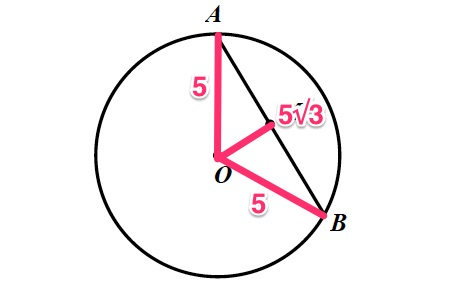Because we know thatis the midpoint of, we can actually cut that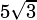in half: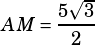and.

We also know that we can always draw a perpendicular bisector from the center of a circle to the midpoint of a chord, so you know that by drawing in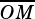, you’re creating two right triangles: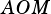and.

Let’s just look at triangle.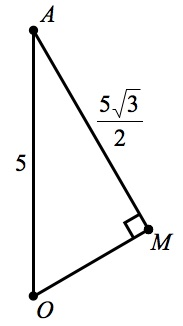At this point, we can certainly use the Pythagorean Theorem to solve, but the presence of the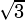should have activated our Spidey-senses from the beginning: this is a 30°-60°-90° triangle! So we can also just see how the numbers we know fit into the knownratio. The hypotenuse in a 30°-60°-90° is double the length of the short leg. Our hypotenuse is 5, so the short leg we seek must be half that:. We know we’re right because the long leg in a30°-60°-90° is the short leg times, and sure enough: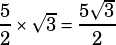. So we have our answer..

Gettin’ Pythaggy wit it: